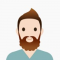## Maximum sum of distinct numbers with LCM as N in C++Ayush Gupta

Updated on 15-Oct-2020 12:13:33

In this problem, we are given a number N. Our task is to create a program to find the maximum sum of distinct numbers with LCM as N in C++.Problem DescriptionHere, we need to find the sum of maximum numbers that have N as the Lowest Common Multiple (LCM).Let’s take ... Read More

## Maximum sum of elements from each row in the matrix in C++Ayush Gupta

Updated on 15-Oct-2020 12:11:37

In this problem, we are given a two matrix mat[][]. Our task is to create a program to find the maximum sum of elements from each row in the matrix in C++.Problem DescriptionHere, we will find the maximum sum by taking one element from each row of the matrix in ... Read More

## Maximum sum such that no two elements are adjacent in C++Ayush Gupta

Updated on 15-Oct-2020 12:07:39

In this problem, we are given an array arr[]. Our task is to create a program to find the Maximum sum such that no two elements are adjacent in C++.Problem DescriptionWe need to find the maximum sum of the sequence from the array such that no 2 numbers from the ... Read More

## Maximum sum such that no two elements are adjacent - Set 2 in C++Ayush Gupta

Updated on 15-Oct-2020 12:05:22

In this problem, we are given an array arr[]. Our task is to create a program to find the Maximum sum such that no two elements are adjacent in C++.Problem DescriptionWe need to find the maximum sum of the sequence from the array such that no 2 numbers from the ... Read More

## Maximum trace possible for any sub-matrix of the given matrix in C++Ayush Gupta

Updated on 15-Oct-2020 12:00:47

In this problem, we are given a two-dimensional array arr[][]. Our task is to create a program to find the Maximum trace possible for any sub-matrix of the given matrix in C++.Problem Descriptionwe need to find the maximum trace for any sub-matrix. The trace is the sum of elements of ... Read More

## Maximum trains for which stoppage can be provided in C++Ayush Gupta

Updated on 15-Oct-2020 11:56:44

In this problem, we are given a number N denoting the number of platforms a station has with two tracks each. And T trains will be passing the station whose arrival and departure time are given. Each train stops at a specific station. Our task is to create a program ... Read More

## Maximum value in an array after m range increment operations in C++Ayush Gupta

Updated on 15-Oct-2020 11:52:25

In this problem, we are given an array arr[] of N elements initialized with 0. Our task is to create a program to find the Maximum value in an array after m range increment operations in C++.Problem DescriptionOn the array, we will perform m range increment operation of the type, ... Read More

## Maximum value K such that array has at-least K elements that are >= K in C++Ayush Gupta

Updated on 09-Oct-2020 09:48:49

In this problem, we are given an array arr. Our task is to create a program to find the Maximum value K such that the array has at-least K elements that are >= K in C++.Problem DescriptionWe need to find a value K, that fulfills the condition that there are ... Read More

## Maximum value of an integer for which factorial can be calculated on a machine in C++Ayush Gupta

Updated on 09-Oct-2020 09:47:58

In this problem, we need to create a program to find Maximum value of an integer for which factorial can be calculated on a machine in C++.Factorial of a number is a huge value, as it is the product of all values preceding it. And C++ can handle large values ... Read More

## Maximum Weight Difference in C++Ayush Gupta

Updated on 09-Oct-2020 09:46:05

In this problem, we are given an array arr[] and a number M. Our task is to create a program to calculate the Maximum Weight Difference in C++.Problem StatementWe will find M elements from the array such that the absolute difference between the sum and the sum of rest elements ... Read More